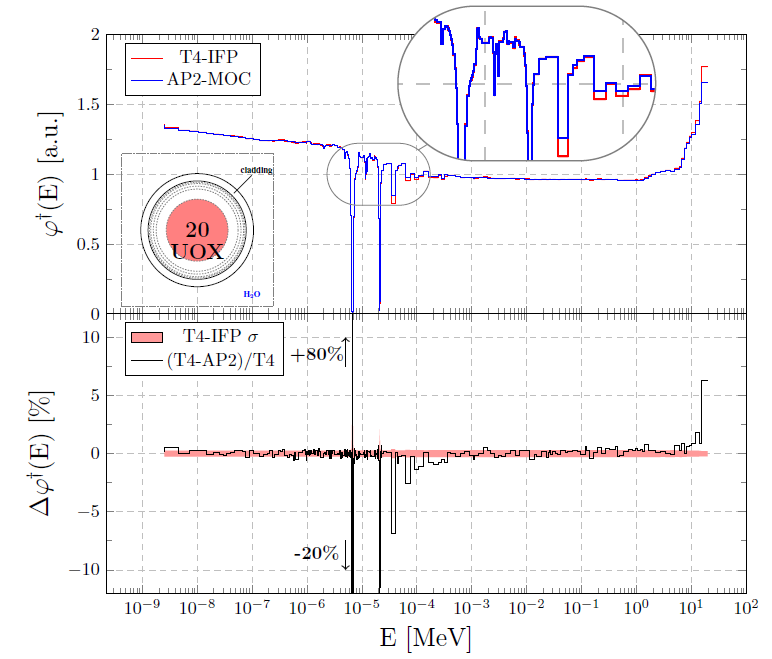# Simulation modes

Publié le 30 novembre 2018

For convenience, particles are organized in batches, which are consecutively simulated according to the simulation mode. The code offers both fixed-source and criticality simulation modes.

In the former, the Monte Carlo code is used to solve the  stationary Boltzmann equation with a given source. The necessary source is imposed by the user (manually, or by importing the initial particle distribution generated by an external code), and particles are then tracked from the source until disappearance. Each batch begins with the same imposed source, hence the name of the simulation mode. Fixed-source mode is typically used for radiation protection and shielding analysis. Fission events can be either treated as sterile captures (in which case fission neutrons are discarded), or explicitly simulated (in which case the secondary fission neutrons are also tracked within the same batch together with the parent particle, and the neutron multiplication factor may be calculated).

In the criticality mode, the Monte Carlo implementation of the power iteration method [1, 2] is used to solve the keff-eigenvalue equation, i.e., to find the fundamental mode and the associated fundamental k-eigenvalue. An initial (guess) neutron source is initially set for the first batch, and the equilibrium neutron distribution corresponding to the keff eigenvalue equation is then sought. To this aim, the power iteration scheme is adopted, which means that at the end of each batch the secondary neutrons generated from fissions are used as a source for the next batch (in between batches, a normalization procedure is applied, as customary). After several such generations when the distribution of fissions has attained convergence, the neutron distribution obeys the fundamental mode of the k-eigenvalue equation. Then, the physical quantities of interest can be scored. The convergence criterion can be either established by the user, or automatically set by the code by resorting to statistical tests so as to assess whether equilibrium has been attained.

The average tallies and the associated variances are estimated from the series of batches, assuming statistical independence of the variables. This assumption is valid for the fixed-source mode, but this is no longer the case for the criticality mode, because of the batch-to-batch correlation induced by the power iteration method. While the average (at the first order) is not affected by this correlation, a bias can be actually observed on the variance estimate, especially for local flux or reaction rates tallies in reactor physics analysis [3, 4]. If the standard power iteration algorithm is applied, the variance computed by the code will be typically underestimated. Moreover, tallies that are expected to be symmetrical might take a non-symmetrical form, because of convergence issues.

Various methods have been proposed so as to overcome this problem: a simple, yet powerful approach consists in grouping batches of particles in packets, and then computing mean and variance on the new grouped series. The difficult point is to assess the right number of packets, large enough to cancel the correlation effect, and small enough to ensure a large number of grouped batches and therefore a reliable estimate of the variance.

Tripoli-4® is also capable of simulating the adjoint neutron flux (the neutron importance function) for the k-eigenvalue problems, by resorting to the Iterated Fission Probability Method [5, 6]. This can be done:

• either in a stand-alone mode, in which case the simulation is actually a fixed-source calculation where the fission neutrons are used as an estimator of the importance of the ancestor neutrons: the resulting importance function is thus the scalar product between the source distribution and the neutron importance . The importance score can be decomposed on a space, angle and energy grid;

• or within a criticality simulation, in which case the importance function is obtained for each active cycle and is then used as a weighting factor in order to produce bi-linear forms involving the forward and adjoint fundamental mode [8, 9]. These bi-linear forms appear for instance in the definitions of the kinetics parameters and other adjoint-weighted scores.Computing the adjoint neutron flux in a UOX fuel assembly: comparison with the deterministic solver Apollo2

A special simulation mode of Tripoli-4® enables all variance reduction and population control techniques to be switched off, thus allowing for fully analog particle transport [10, 11]. This option is available for neutrons and photons separately. For neutrons, specifically, Tripoli-4® has been coupled to the fission models available in the FREYA  and FIFRELIN  codes, in order to explicitly take into account the neutron multiplicity and the correlations due to fission events, with application to coincidence detection.

​References

 E. E. Lewis, W.F. Jr. Miller, Computational Methods of Neutron Transport, J. Wiley and Sons (1984).

 I. Lux, L. Koblinger, Monte Carlo particle transport methods : Neutron and photon calculations, CRC Press, Boca Raton (1991).

 F.-X. Hugot, in Proceedings of Physor2008, Interlaken, Switzerland (2008).

 E. Dumonteil et al., Ann. Nucl. Energy 63, 612-618 (2014).

 Y. Nauchi, T. Kameyama, J. Nucl. Sci. Technol. 47, 977 (2010).

 B.C. Kiedrowski, F.B. Brown, P.P.H. Wilson, Nucl. Sci. Eng. 68, 226 (2011).

 N. Terranova, G. Truchet, I. Zmijarevic, A. Zoia, Ann. Nucl. Energy 114, 136-148 (2018).

 G. Truchet, P. Leconte, A. Santamarina, F. Damian, A. Zoia, Ann. Nucl. Energy 81, 17-26 (2015).

 N. Terranova, A. Zoia, Ann. Nucl. Energy 108, 57-66 (2017).

 O. Petit, E. Dumonteil, ANS RPSD-2014, 18th Topical Meeting of Radiation Protection & Shielding Division of ANS, Knoxville, USA (2014).

 O. Petit, E. Dumonteil, Nucl. Technol. 192, 259-263 (2015).

 J.M. Verbeke, O Petit, Nucl. Sci. Eng. 183, 214-228 (2016).

 O. Petit, C. Jouanne, O. Litaize, O. Serot, A. Chebboubi, Y. Pénéliau, in Proceedings of ICRS-13 & RPSD-2016, Paris, France, 2016. EPJ Web of Conferences 153, 06003 (2017).